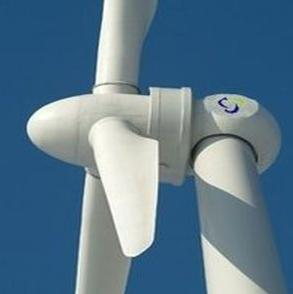# Calculation of Wind Power

There are many complicated calculations and equations involved in understanding and constructing wind turbine generators however the layman need not worry about most of these and should instead ensure they remember the following vital information:1) The power output of a wind generator is proportional to the area swept by the rotor – i.e. double theswept area and the power output will also double.

2) The power output of a wind generator is proportional to the cube of the wind speed – i.e. double the wind speed and the power output will increase by a factor of eight (2 x 2 x 2)!

If you are not mathematically minded you can quit now, however it is well worth trying to understand what is going on here.

### The Power of Wind

Wind is made up of moving air molecules which have mass – though not a lot. Any moving object with mass carries kinetic energy in an amount which is given by the equation:

Kinetic Energy = 0.5 x Mass x Velocity2

where the mass is measured in kg, the velocity in m/s, and the energy is given in joules.

Air has a known density (around 1.23 kg/m3 at sea level), so the mass of air hitting our wind turbine (which sweeps a known area) each second is given by the following equation:

Mass/sec (kg/s) = Velocity (m/s) x Area (m2) x Density (kg/m3)

And therefore, the power (i.e. energy per second) in the wind hitting a wind turbine with a certain swept area is given by simply inserting the mass per second calculation into the standard kinetic energy equation given above resulting in the following vital equation:

Power = 0.5 x Swept Area x Air Density x Velocity3

where Power is given in Watts (i.e. joules/second), the Swept area in square metres, the Air density in kilograms per cubic metre, and the Velocity in metres per second.

### Read World Wind Power Calculation

The world’s largest wind turbine generator has a rotor blade diameter of 126 metres and so the rotors sweep an area of PI x (diameter/2)2 = 12470 m2! As this is an offshore wind turbine, we know it is situated at sea-level and so we know the air density is 1.23 kg/m3. The turbine is rated at 5MW in 30mph (14m/s) winds, and so putting in the known values we get:

Wind Power = 0.5 x 12,470 x 1.23 x (14 x 14 x 14)
…which gives us a wind power of around 21,000,000 Watts. Why is the power of the wind (21MW) so much larger than the rated power of the turbine generator (5MW)? Because of the Betz Limit, and inefficiencies in the system.